## Tuesday, April 23, 2019

### Seasonal Unit Root Tests

Author and guest post by Nicolas Ronderos

In this blog entry we will offer a brief discussion on some aspects of seasonal non-stationarity and discuss two popular seasonal unit root tests. In particular, we will cover the Hylleberg, Engle, Granger, and Yoo (1990) and Canova and Hansen (1995) tests and demonstrate practically using EViews how the latter can be used to detect the presence of seasonal unit roots in a US macroeconomic time series. All files used in this exercise can be downloaded at the end of the entry.

### Deterministic vs Stochastic Seasonality

When we talk about the concept of seasonality in time series, we usually refer to the idea of "... systematic, although not necessarily regular, intra-year movement caused by changes of the weather, the calendar, and timing of decisions..." (Hans Franses). Naturally, macroeconomic data observed with high periodicity (sampled more than once a year) usually exhibit this behavior.

Seasonality can be modelled in two ways: deterministically or stochastically. The former arises form systematic cycles such as calendar effects or climatic phenomena and can be removed from data by the seasonal adjustment procedures -- in other words, by including seasonal dummy variables. Formally, this implies deterministic seasonality evolves as:

$$y_{t} = \mu + \sum_{s=1}^{S-1}\delta_{s}D_{s,t} + e_{t}$$ where $S$ is the total number of period cycles, $D_{s,t}$ are seasonal dummy variables which equal 1 in season $s$ and 0 otherwise, and $e_{t}$ are the usual innovations. For example, in the case of quarterly data $(S=4)$, one could postulate that seasonality evolves as:

$$y_{t} = 15 - D_{1,t} - 4D_{2,t} - 6D_{3,t} + e_{t}$$ The process is visualized below: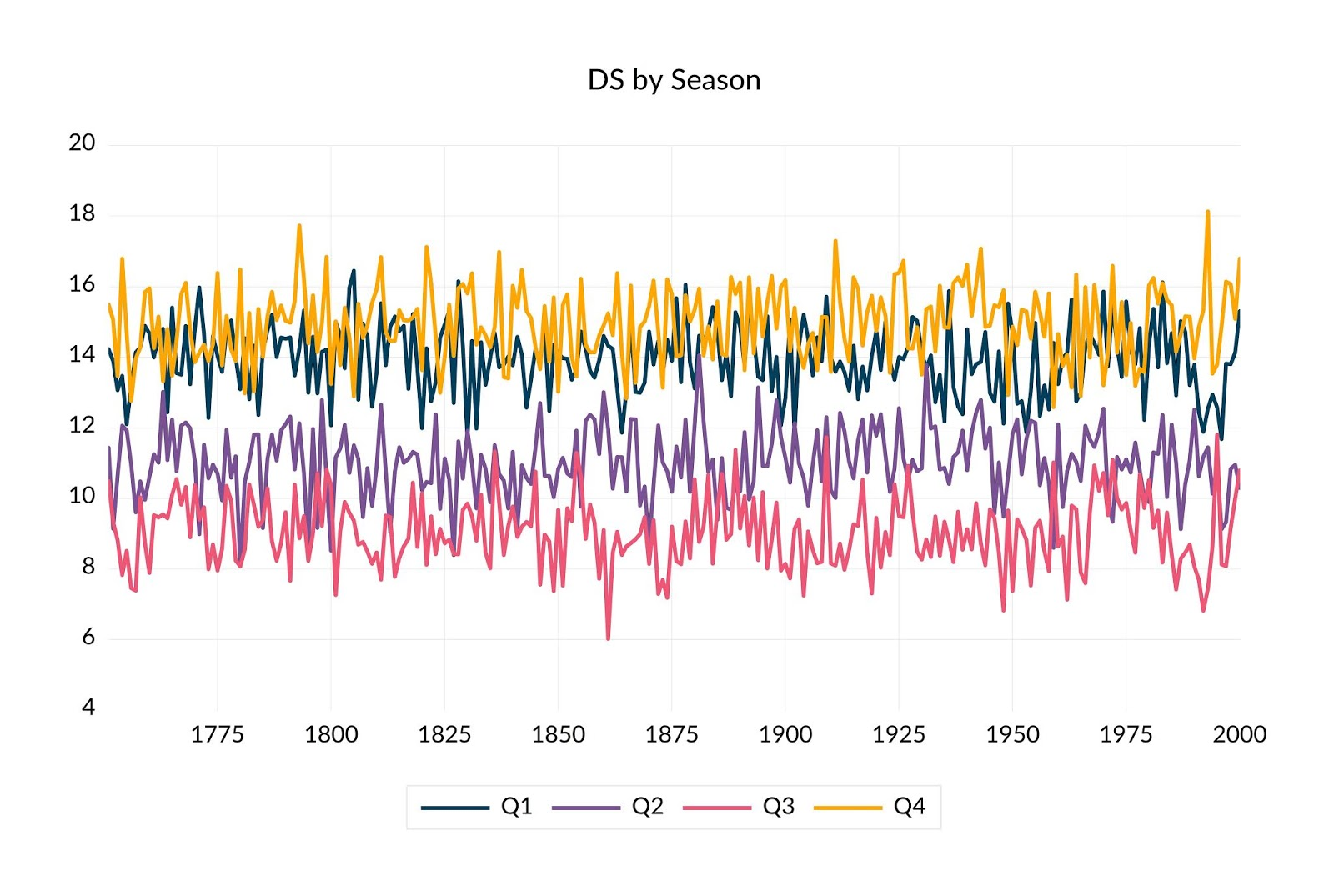Figure 1: Deterministic Seasonality

Notice here that the optimal $h$-period ahead forecast of $y_{t}$ in season $s$, is given by:

$$\widehat{y}_{S(t+h)-s} = \widehat{\mu} + \widehat{\delta}_{s}$$ where $s = S-1, \ldots, 0$. In other words, the optimal forecast of $y_{t}$ in season $s$ is the same at each future point in time for said season. It is precisely this property which formalizes the notion of systematic cyclicality.

On the other hand, stochastic seasonality describes nearly systematic cycles which evolve as seasonal ARMA$(p,q)$ processes of the form:

$$(1 - \eta_{1}L^{S} - \eta_{2}L^{2S} - \ldots - \eta_{p}L^{pS})y_{t} = (1 + \xi_{1}L^{S} + \xi_{2}L^{2S} + \ldots + \xi_{q}L^{qS})e_{t}$$ where $L$ denotes the usual lag operator. In particular, when $p = 1$ and $q = 0$, the seasonal AR(1) model with $\eta_{1} = 0.75$ is visualized as follows: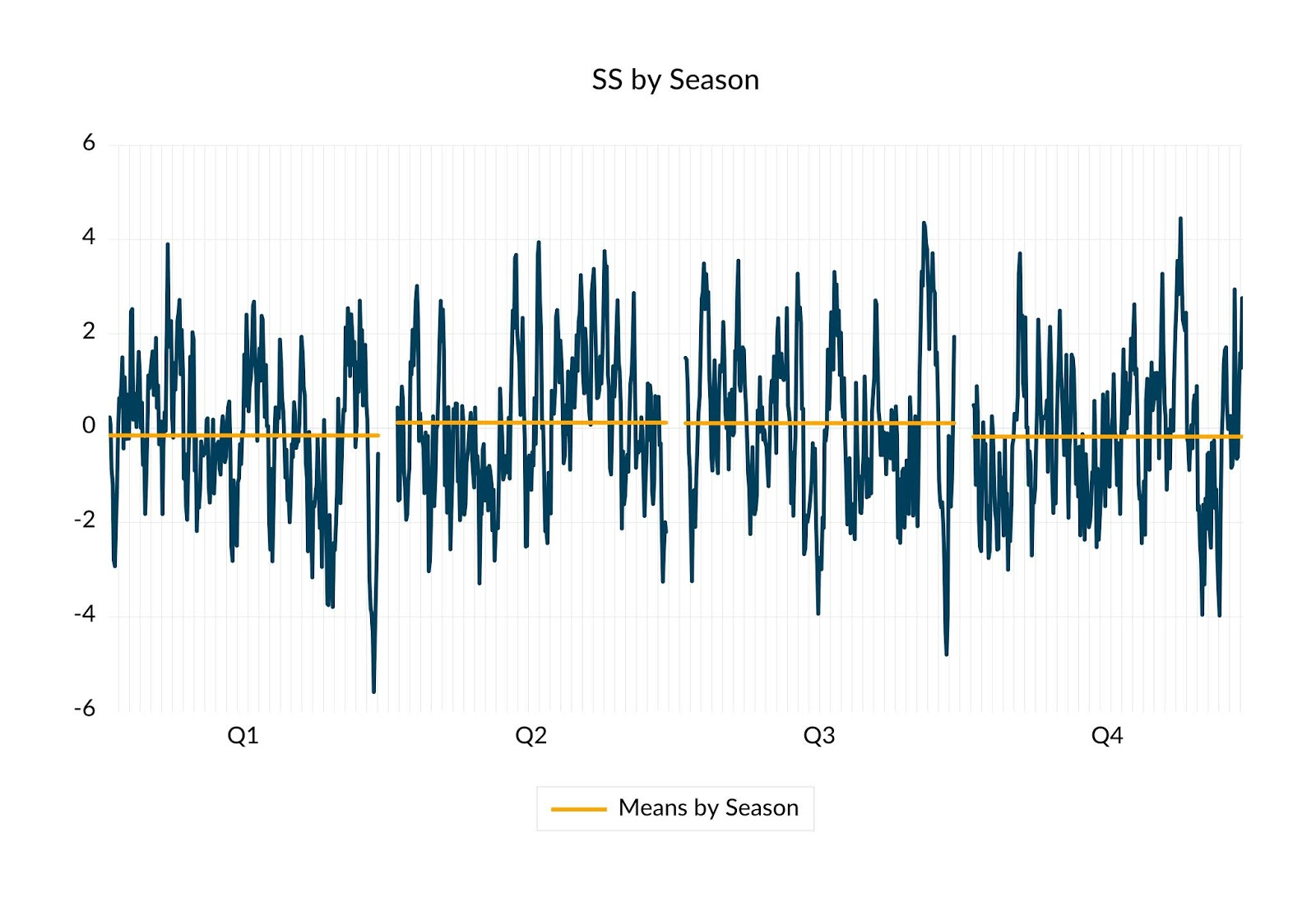Figure 2: Stochastic Seasonality

Unlike the deterministic seasonal model however, the $h$-period ahead forecast of the stochastic seasonal model is not constant. In particular, for the seasonal AR(1) model, the forecast $h$-periods ahead is given by:

$$\widehat{y}_{S(t+h)-s} = \widehat{\eta}_{1}^{h}y_{St-s}$$ In other words, the forecast in any given season is a function of past data values, and is therefore considered to be stochastic.

So how does one identify whether a series exhibits deterministic or stationary seasonality? One useful tool is the periodogram which produces a decomposition of the dominant frequencies (cycles) of a time series. As it turns out, there are at most $S$ frequencies in a time series exhibiting $S$ period cycles. Formally, these are identified in conjugate pairs as follows:

$$\omega \in \left\{0, \left(\frac{2\pi}{S}, 2\pi-\frac{2\pi}{S}\right), \left(\frac{4\pi}{S}, 2\pi-\frac{4\pi}{S}\right), \ldots, \pi \right\}$$ if $S$ is even, and

$$\omega \in \left\{0, \left(\frac{2\pi}{S}, 2\pi-\frac{2\pi}{S}\right), \left(\frac{4\pi}{S}, 2\pi-\frac{4\pi}{S}\right), \ldots, \left(\frac{\lfloor S/2 \rfloor\pi}{S}, 2\pi-\frac{\lfloor S/2\rfloor\pi}{S}\right) \right\}$$ if $S$ is odd.

Thus, given a stationary time series with $S$ period cycles, we expect the periodogram to protrude at the non-zero frequencies. In particular, we present the periodogram for deterministic and stochastic seasonal processes below: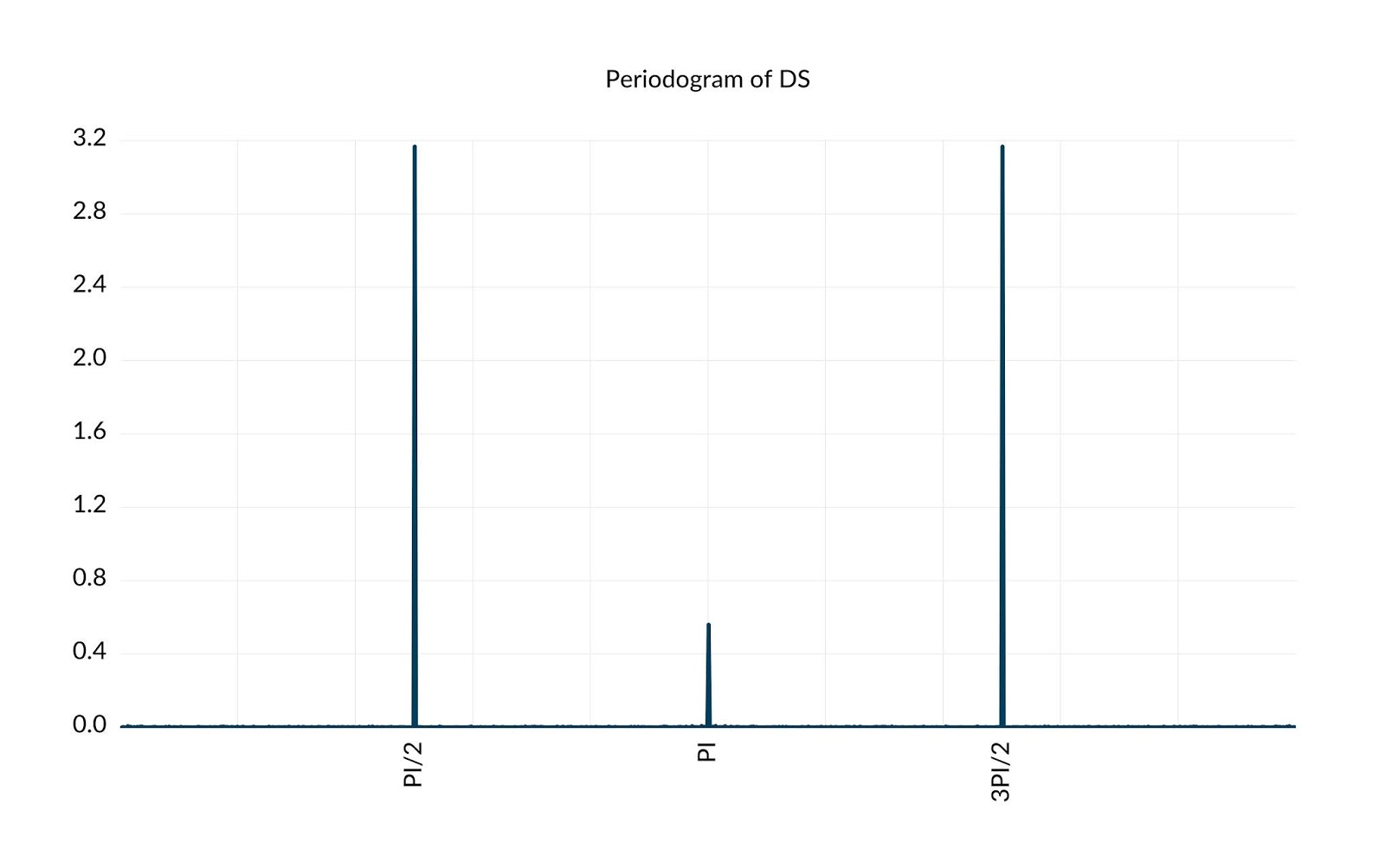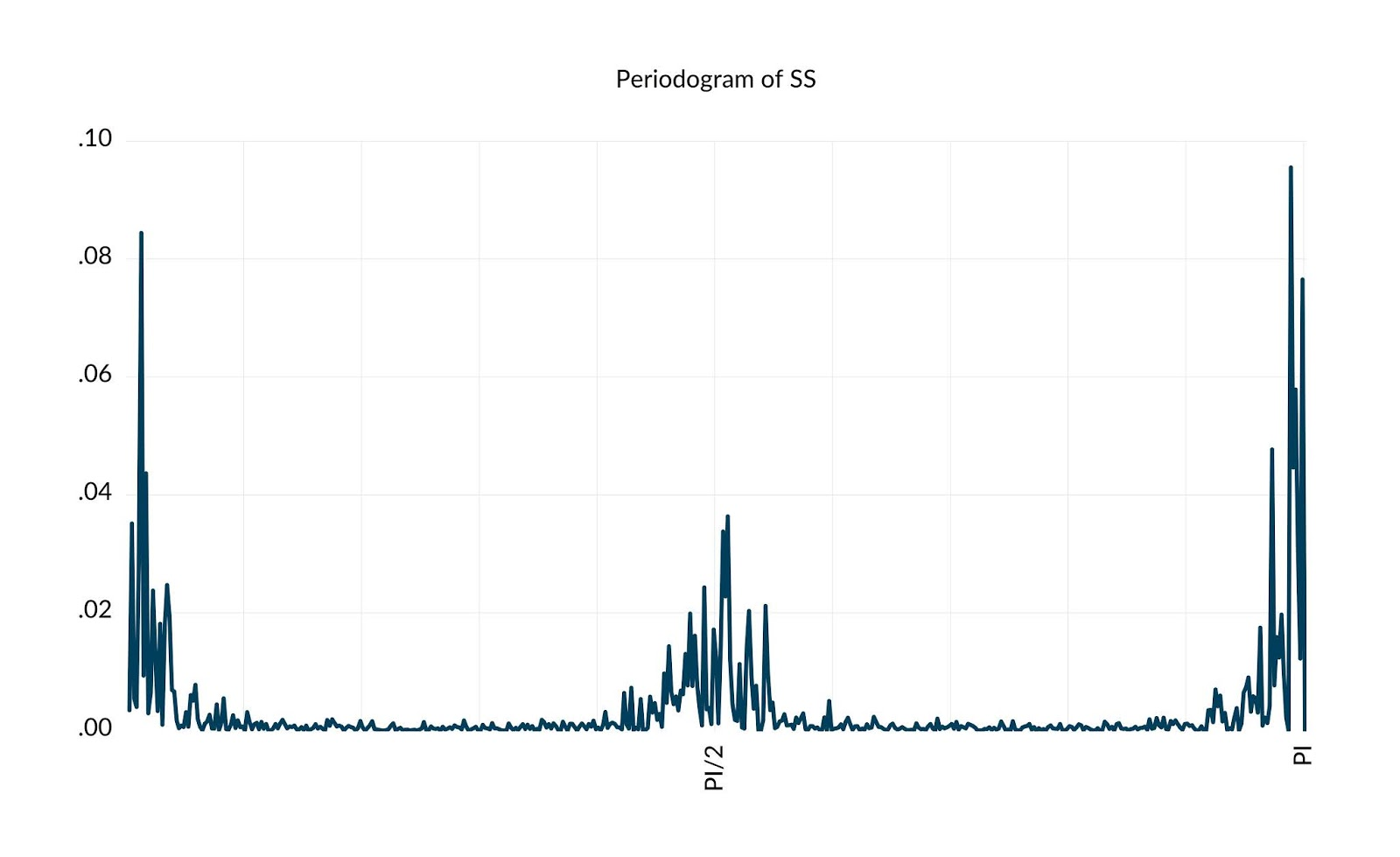Figure 3A: Deterministic Seasonality Periodogram Figure 3B: Stochastic Seasonality Periodogram
We can see from the periodograms that the spectrum of deterministic seasonal processes exhibits sharp peaks at the seasonal frequencies $\omega$, whereas that of stochastic seasonal processes exhibits a window of sharp peaks centered around seasonal frequencies $\omega$. In case of stochastic seasonality, the fact that the spectrum spreads around principal frequencies and is not a single peak reaffirms the notion that cycles are stochastically distributed around said frequencies.

### Seasonal Unit Roots

A particularly important form of stochastic seasonality manifests in the form of unit roots at some or all of the frequencies $\omega$. In particular, consider the following process:

$$y_{t} = \eta y_{t-S} + e_{t}$$ and note that the characteristic equation associated with the process is defined as:

\begin{align} 1 - \eta z^{S} = 0 \quad \text{or} \quad z^{S} = 1/\eta \label{eq1} \end{align} Analogous to the case of classical unit root processes, when $|\eta|=1$ or $|z| = 1^{1/S} = 1$, $y_{t}$ is in fact non-stationary. In contrast to the classical unit root case however, $y_{t}$ can possess not one, but upto $S$ unique unit roots. To see this, note that any complex number $z = a + ib$ can be written in polar form as:

$$z = \sqrt{a^{2} + b^{2}}(\cos(\theta) + i\sin(\theta)) = r(\cos(\theta) + i\sin(\theta))$$ where $r = |z|$ is called the magnitude of $z$, but is also the radius of the circle in polar coordinates. Accordingly, when $|\eta | = 1$ or $|z|=1$, $z$ lies on a circle with radius $r = 1$. In other words, $y_{t}$ is a unit root process. Next, recall Euler's formula:

$$e^{ix} = \cos(x) + i \sin(x)$$ Clearly, any complex number $z$ with magnitude $r=1$ satisfies Euler's formula. In other words, $z = e^{i\theta}$. Since Euler's formula also implies that:

$$e^{2\pi i k} = 1 \quad \text{for} \quad k=0,1,2,\ldots$$ when $\eta=1$ or $|z|=1$, the characteristic equation \eqref{eq1} can be expressed as:

\begin{align*} z = e^{i\omega} &= 1^{1/S} \notag\\ &= (e^{2\pi i k})^{1/S}\notag\\ &= e^{\frac{2\pi i k}{S}} \end{align*} where the relations above evidently hold for all $k=0,1,2,\ldots, S-1$ since the solutions begin to cycle when $k \geq S$. Now, taking logarithms of both sides, it is clear that:

\begin{align} \omega = \frac{2\pi k}{S} \quad \text{for} \quad k=0,1,2,\ldots, S-1 \label{eq2} \end{align} In other words, the characteristic equation \eqref{eq1} has $S$ unique solutions identified by the $S$ relationships in \eqref{eq2}. These solutions are equally (by $2\pi k/S$ degrees) spaced on the unit circle, with two real solutions associated with $\omega = 0$ and $\omega = \pi$, and the remaining $S-2$ imaginary solutions organized in harmonic pairs.

Thus, when we identify $S$ with a temporal frequency, namely a week, month, quarter, and so on, the problem of identifying roots of the characteristic equation \eqref{eq1} extends the classical unit root literature in which $S=1$ (or annual frequency), to that of identifying $S > 1$ possible roots on the unit circle.

In fact, like the classical unit-root literature in which unchecked unit roots are known to have severe inferential consequences, the presence of unit roots at seasonal frequencies can also give rise to similar inferential inaccuracies and concerns. Accordingly, identifying the presence of unit roots at one or more seasonal frequencies is the subject of the battery of tests known as seasonal unit root tests.

### Seasonal Unit Root Tests

Historically, the first test for a seasonal unit root was proposed by Dickey, Hasza and Fuller (1984) (DHF). In its simplest form, the test is based on running the regression:

$$(1-L^{S})y_{t} = \eta y_{t-s} + e_{t}$$ and testing the null hypothesis $H_{0}: \eta = 0$ against the one-sided alternative $H_{A}: \eta < 0$. The test is carried out using the familiar Student's-$t$ statistic on statistical significance for $\eta$, and analogous to the classic augmented Dickey-Fuller (ADF) test, exhibits a non-standard asymptotic distribution under the null. Nevertheless, the DHF test is very restrictive. Whereas the test imposes the existence of a unit root at all $S$ seasonal frequencies simultaneously, in reality, a process may exhibit a seasonal unit root at some seasonal frequencies but not others.

#### HEGY Seasonal Unit Root Test

To correct for the shortcomings of the DHF test, Hylleberg, Engle, Granger and Yoo (1990) (HEGY) proposed a test for the determination of unit roots at each of the $S$ seasonal frequencies individually, or collectively. In particular, following the notation in Smith and Taylor (1999), in its simplest form, the HEGY test is based on regressions of the form:

\begin{align*} (1-L^{s})y_{St-s} &= \mu + \pi_{0}L\left(1 + L + \ldots + L^{S-1}\right)y_{St-s}\\ &+ L\sum_{k=1}^{S^{\star}}\left( \pi_{k,1}\sum_{j=0}^{S-1}\cos\left((j+1)\frac{2\pi k}{S}\right)L^{j} - \pi_{k,2}\sum_{j=0}^{S-1}\sin\left((j+1)\frac{2\pi k}{S}\right)L^{j} \right)y_{St-s}\\ &+ \pi_{S/2}L\left(1 - L + L^{2} - \ldots - L^{S-1}\right)y_{St-s} + e_{t}\\ &\equiv \mu + \pi_{0}y_{St-s-1, 0} + \sum_{k=1}^{S^{\star}}\pi_{k,1}y_{St-s-1,k,1} + \sum_{k=1}^{S^{\star}}\pi_{k,2}y_{St-s-1,k,2} + \pi_{S/2}y_{St-s-1, S/2} +e_{t} \end{align*} where $S^{\star} = (S/2) - 1$ if $S$ is even and $S^{\star} = \lfloor S/2 \rfloor$ if $S$ is odd, and as before, $s = S-1, \ldots, 1, 0$.

In particular, when data is quarterly with $S=4$ and therefore $S^{\star} = 1$, then:

\begin{align*} y_{4t-s, 0} &= (1+L+L^{2}+L^{3})y_{4t-s}\\ y_{4t-s, 1,1} &= -L(1-L^{2})y_{4t-s}\\ y_{4t-s, 1,2} &= -(1-L^{2})y_{4t-s}\\ y_{4t-s, 2} &= -(1-L+L^{2}-L^{3})y_{4t-s} \end{align*} Here, $y_{4t-s, 0}$ is in fact the series $y_{4t-s}$ filtered by the 0 frequency filter, $y_{4t-s, 1,1}$ is the series $y_{4t-s}$ filtered by the $\pi/2$ frequency filter, $y_{4t-s, 1,2}$ is the series $y_{4t-s}$ filtered by the $3\pi/2$ frequency filter, and $y_{4t-s, 2}$ is the series $y_{4t-s}$ filtered by the $\pi$ frequency filter.

To visualize the frequency filters, consider the spectral filter functions associated with each of the processes above. The latter are computed as $|\phi(e^{i\theta})|$ where $\phi(\cdot)$ is the lag polynomial applied to $y_{St-s}$, and $\theta \in [0, 2\pi)$. For instance, in case of quarterly data, the 0 frequency filter is computed as $|1 + e^{i\theta} + e^{i2\theta} + e^{i3\theta} + e^{i4\theta}|$, and so on.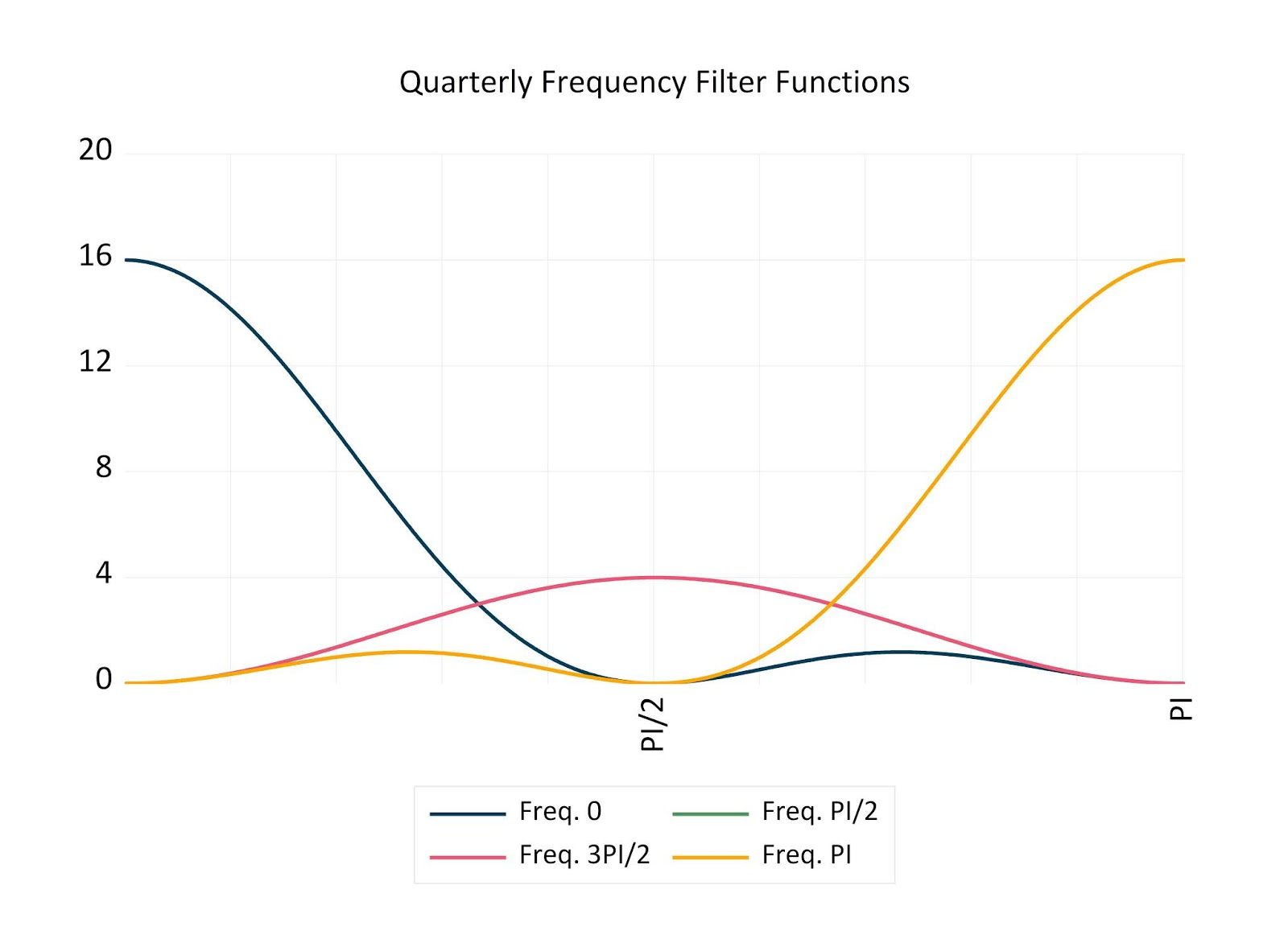Figure 4: HEGY Seasonal Filters

Like the DHF test, the HEGY test also reduces to verifying parameter significance in the regression equation. Nevertheless, in contrast to DHF, HEGY tests can detect isolated effect of each seasonal frequency independently. In the case of quarterly data, for instance, a $t-$test on coefficient significance for $\pi_{1} = 0$ is in fact a test for a unit root in the $\omega = 0$ frequency, a $t-$test on coefficient significance for $\pi_{2} = 0$ is a test for the presence of a unit root at the $\omega = \pi$ frequency, and an $F-$test for the joint parameter significance of $\pi_{1,1} = 0$ and $\pi_{1,2} = 0$, is in fact a joint test for the presence of a unit root at the harmonic conjugate pair of frequencies $(\pi/2, 3\pi/2)$.

It should also be noted here that while we have focused on the simplest form, the HEGY test can accommodate various deterministic specifications in the form of seasonal dummies, constants, and trends. Moreover, in the presence of serial correlation in the innovation process, the HEGY test can also be augmented with lags of the dependent variable as additional regressors to the principal equation presented above, in order to mitigate the effect.

In fact, the HEGY test is very similar to the ADF test which is effectively a unit root test at the 0-frequency alone. Whereas the latter proceeds as a regression of a differenced series against its lagged level, the former proceeds as a regression of a seasonally differenced series against the lagged levels at each of the constituent seasonal frequencies. In this regard, the HEGY test is considered an extension of the ADF test in the direction of non-zero frequencies. As such, it also suffers from the same shortcomings as the ADF test, and can exhibit low statistical power when the individual frequencies are in fact stationary, but exhibit near-unit root behaviour.

#### Canova-Hansen Seasonal Unit Root Test

One response to the low power of ADF tests in the presence of near unit root stationarity was the test of Kwiatkowski, Phillips, Schmidt, and Shin (1992) (KPSS), which is in fact a test for stationarity at the 0-frequency alone. The analogous development in the seasonal unit root literature was the test of Canova and Hansen (1995) (CH). Like the KPSS test, the CH test is also a test for stationarity but extends to non-zero seasonal frequencies.

The idea behind the CH test is to suppose that seasonality manifests in the process mean. In other words, given a process $y_{t}$, if seasonal effects are present, then $y_{t}$ will exhibit a seasonally dependent average. Traditionally, this is formalized using seasonal dummy variables as:

$$y_{t} = \sum_{s=0}^{S-1}\delta_{s}D_{s,t} + e_{t}$$ Nevertheless, it is well known that an equivalent representation using discrete Fourier expansions exists in terms of sine and cosine functions. In particular,

$$y_{t} = \sum_{k=0}^{S^{\star}}\left(\delta_{k,1}\cos\left(\frac{2\pi k t}{S}\right) + \delta_{k,2}\sin\left(\frac{2\pi k t}{S}\right)\right) + e_{t}$$ where $S^{\star}$ was defined earlier, and $\delta_{k,1}$ and $\delta_{k,2}$ are referred to as spectral intercept coefficients. In either case, the expression can be expressed in vector notation as follows:

\begin{align} y_{t} = \pmb{Z}_{t}^{\top}\pmb{\gamma}_{t} + e_{t} \label{eq3} \end{align} where $\pmb{Z}_{t} = \left(1, \pmb{z}_{1,t}^{\top}, \ldots, \pmb{z}_{S^{\star},t}^{\top} \right)$ (or $\pmb{Z}_{t} = \left(1, D_{1,t}, \ldots, D_{S-1,t}\right)$) and $\pmb{\gamma}_{t} = \left(\gamma_{1,t}, \ldots, \gamma_{S,t}\right)$ is a an $S\times 1$ vector of coefficients, and $\pmb{z}_{k,t} = \left(\cos\left(\frac{2\pi k t}{S}\right), \sin\left(\frac{2\pi k t}{S}\right)\right)$ for $j=1,\ldots, S^{\star}$, with the convention $\pmb{z}_{S^{\star},t} \equiv \cos(\pi t) = (-1)^{t}$ when $S$ is even.

Next, to distinguish between stationary and non-stationary seasonality, CH assume that the coefficient vector $\pmb{\gamma}_{t}$ evolves as the following AR(1) model:

\begin{align*} \pmb{\gamma}_{t} &= \pmb{\gamma}_{t-1} + u_{t}\\ u_{t} &\sim IID(\pmb{0}, \pmb{G})\\ \pmb{G} &= \text{diag}(\theta_{1}, \ldots, \theta_{S}) \end{align*} Observe that when $\theta_{k} > 0$, then $\gamma_{k,t}$ follows a random walk. On the other hand, when $\theta_{k} = 0$, then $\gamma_{k,t} = \gamma_{k, t-1} = \gamma_{k}$, a fixed constant for all $t$. In other words, when $\theta_{k} > 0$, the process $y_{t}$ exhibits a seasonal unit root at the harmonic frequency pair $(\frac{2\pi k}{S}, 2\pi - \frac{2\pi k}{S})$ for $1\leq k < \lfloor S/2 \rfloor$, and the frequency $\frac{2\pi k}{S}$ if $k=0$ or $k = \lfloor S/2 \rfloor$. In this regard, to test the null hypothesis that $y_{t}$ exhibits at most deterministic seasonality at certain (possibly all) frequencies, against the alternative hypothesis that $y_{t}$ exhibits a seasonal unit root at certain (possibly all) frequencies, define $\pmb{A}_1$ and $\pmb{A}_2$ as mutually orthogonal, full column-rank, $(S \times a_1)-$ and $(S \times a_2)$-matrices which respectively constitute $1 \leq a_1 \leq S$ and $a_2 = S - a_1$ sub-columns from the order-$S$ identity matrix $\pmb{I}_s$.

For instance, if one wishes to test whether a seasonal unit root exists at frequency $\pi$, one would set $\pmb{A}_{1} = (0,\ldots, 0,1)^{\top}$. Alternatively, if testing for a seasonal unit root at the frequency pair $\left(\frac{2\pi}{S}, 2\pi - \frac{2\pi}{S}\right)$, then one would set:

$$\pmb{A}_{1} = \begin{bmatrix} 0 & 0 \\ 1 & 0 \\ 0 & 1 \\ 0 & 0 \\ \vdots & \vdots \\ 0 & 0 \end{bmatrix}$$ Note further that one can further rewrite \eqref{eq3} as follows:

$$y_{t} = \pmb{Z}_{t}^{\top}\pmb{A}_{1}\pmb{A}_{1}^{\top}\pmb{\gamma}_{t} + \pmb{Z}_{t}^{\top}\pmb{A}_{2}\pmb{A}_{2}^{\top}\pmb{\gamma}_{t} + e_{t}$$ Next, define $\pmb{\Theta} = \left(\theta_{1}, \ldots, \theta_{S}\right)^{\top}$ and observe that the CH hypothesis battery reduces to:

\begin{align*} H_{0}: \text{}\pmb{A}_{1}^{\top}\pmb{\Theta} = \pmb{0}\\ H_{A}: \text{}\pmb{A}_{1}^{\top}\pmb{\Theta} > 0 \end{align*} where in addition to $H_{0}$, it is implicitly maintained that $H_{M}:\text{} \pmb{A}_{2}^{\top}\pmb{\Theta} = \pmb{0}$. In particular, notice that when both $H_{0}$ and $H_{M}$ hold, equation \eqref{eq3} reduces to:

\begin{align} y_{t} = \pmb{Z}_{t}^{\top}\pmb{\gamma} + e_{t} \label{eq4} \end{align} where $\pmb{\gamma}$ is now constant across time. In other words, $y_{t}$ exhibits at most deterministic (stationary) seasonality. In this regard, holding $H_{M}$ implicitly true, Canova and Hansen (1995) propose a consistent test for $H_{0}$ versus $H_{A}$, using the statistic:

\begin{align*} \mathcal{L} = T^{-2} \text{tr}\left(\left(\pmb{A}_{1}^{\top}\widehat{\pmb{\Omega}}\pmb{A}_{1}\right)^{-1}\pmb{A}_{1}^{\top}\left(\sum_{t=1}^{T}\widehat{F}_{t}\widehat{F}_{t}^{\top}\right)\pmb{A}_{1}^{\top}\right) \end{align*} where $\text{tr}(\cdot)$ is the trace operator, $\widehat{e}_{t}$ are the OLS residuals from regression \eqref{eq4}, $\widehat{F}_{t} = \sum_{t=1}^{T} \widehat{e}_{t}\pmb{Z}_{1,t}$, and the HAC estimator

$$\widehat{\pmb{\Omega}} = \sum_{j=-T+1}^{T-1}\kappa\left(\frac{j}{h}\right)\widehat{\pmb{\Gamma}}(j)$$ Above, $\kappa(\cdot)$ is the kernel function, $h$ is the bandwidth parameter, and $\widehat{\pmb{\Gamma}}(j)$ is the autocovariance (at level $j$ ) estimator

$$\widehat{\pmb{\Gamma}}(j) = T^{-1} \sum_{t=j+1}^{T} \widehat{e}_{t}\pmb{Z}_{t}\widehat{e}_{t-j}\pmb{Z}_{t-j}^{\top}$$ Naturally, we reject the null hypothesis when $\mathcal{L}$ is larger than some critical value which depends on the rank of $\pmb{A}_{1}$.

#### Unattended Unit Roots

A well-known problem with the CH test concerns the issue of unattended unit roots. In particular, CH tests the null hypothesis $H_{0}$ while imposing $H_{M}$, where the latter lies in the complementary space to that generated by the former. In practice however, one does not know which spectral frequency exhibits a unit root. If one did know, the exercise of testing for their presence would be nonsensical. In this regard, if $H_{0}$ is imposed but $H_{M}$ is violated, then, Taylor (2003) shows that the CH test is severely undersized. To overcome the shortcoming, Taylor (2003) suggests filtering the regression equation \eqref{eq3} to reduce the order of integration at all spectral frequencies identified in $\pmb{A}_{2}$. In particular, consider the filter:

$$\nabla_{2} = \frac{1 - L^{S}}{\nabla_{1}}$$ where $\nabla_{1}$ reduces, by one, the order of integration at each frequency identified in $\pmb{A}_{1}$. For instance, if $\pmb{A}_{1}$ identifies the 0-frequency, then $\nabla_{1} = (1 - L)$ and $\nabla_{2} = \frac{1-L^{S}}{1-L} = 1 + L + \ldots + L^{S-1}$. Alternatively, if $\pmb{A}_{1}$ identifies the harmonic frequency pair $\left(\frac{2\pi k}{S}, 2\pi - \frac{2\pi k}{S}\right)$, then $\nabla_{1} = 1 - 2\cos\left(\frac{2\pi k}{S}\right)L + L^{2}$, and so on. Accordingly, if we assume $\pmb{\gamma}_{t} = \pmb{\gamma}_{t-1} + u_{t}$, it is clear that $\nabla_{2}y_{t}$ will not admit unit root behaviour at any of the frequencies identified in $\pmb{A}_{2}$ and the maintained hypothesis $H_{M}$ will hold. See Taylor (2003) and Busetti and Taylor (2003) for further details.

Furthermore, since $\nabla_{2}$ acts only on frequencies identified in $\pmb{A}_{2}$, it can also be formally shown that the regressors $\nabla_{2}\pmb{Z}_{t}^{\top}\pmb{A}_{1}$ span a space identical to the space spanned by $\pmb{Z}_{t}^{\top}\pmb{A}_{1}$. Accordingly, the strategy in Taylor (2003) is to run the regression:

\begin{align*} \nabla_{2}y_{t} &= \nabla_{2}\pmb{Z}_{t}^{\top}\pmb{A}_{1}\pmb{A}_{1}^{\top}\pmb{\gamma}_{t} + \nabla_{2}\pmb{Z}_{t}^{\top}\pmb{A}_{2}\pmb{A}_{2}^{\top}\pmb{\gamma}_{t} + \nabla_{2}e_{t} \\ &= \pmb{Z}_{t}^{\top}\pmb{A}_{1}\pmb{A}_{1}^{\top}\pmb{\gamma}_{t} + e_{t}^{\star} \end{align*} where $e_{t}^{\star} = \nabla_{2}\pmb{Z}_{t}^{\top}\pmb{A}_{2}\pmb{A}_{2}^{\top}\pmb{\gamma}_{t} + \nabla_{2}e_{t}$. Naturally, the modified test statistic is now given by:

\begin{align*} \mathcal{L}^{\star} = T^{-2} \text{tr}\left(\left(\pmb{A}_{1}^{\top}\widehat{\pmb{\Omega}}^{\star}\pmb{A}_{1}\right)^{-1}\pmb{A}_{1}^{\top}\left(\sum_{t=1}^{T}\widehat{F}_{t}^{\star}\widehat{F}_{t}^{\star^{\top}}\right)\pmb{A}_{1}^{\top}\right) \end{align*} where $\widehat{F}_{t}^{\star} = \sum_{t=1}^{T} \widehat{e}_{t}^{\star}\pmb{Z}_{1,t}$ and $\widehat{\pmb{\Omega}}^{\star}$ is computed analogous to $\widehat{\pmb{\Omega}}$ upon replacing $\widehat{e}_{t}$ with $\widehat{e}_{t}^{\star}$.

### Seasonal Unit Root Test in EViews

Starting with version 11 of EViews, a battery of tests aimed at diagnosing unit roots in the presence seasonality are now supported natively. These tests include the most famous Hylleberg, Engle, Granger, and Yoo (1990) (HEGY) test as well its Smith and Taylor (1999) likelihood ratio variant, the Canova and Hansen (1995) (CH) test, and the Taylor (2005) variance ratio test.

Here, we will apply the HEGY and CH tests to detect the presence of seasonal unit roots in quarterly U.S. government consumption expenditures and gross investment data running from 1947 to 2018. We have named the series object containing the data as USCONS. The latter can either be opened from the workfile associated with this blog, or by running a fetch procedure to grab the data directly from the FRED database. In case of the latter, in EViews, issue the following commands in the command window:

    wfcreate q 1947q1 2018q4
fetch(d=fred) NA000333Q
rename NA000333Q uscons

We begin with a plot of the data. To do so, double click on a USCONS in the workfile to open the series object. Next, click on View/Graph.... This will open a graph options window. We will stick with the defaults so click on OK. The output is reproduced below.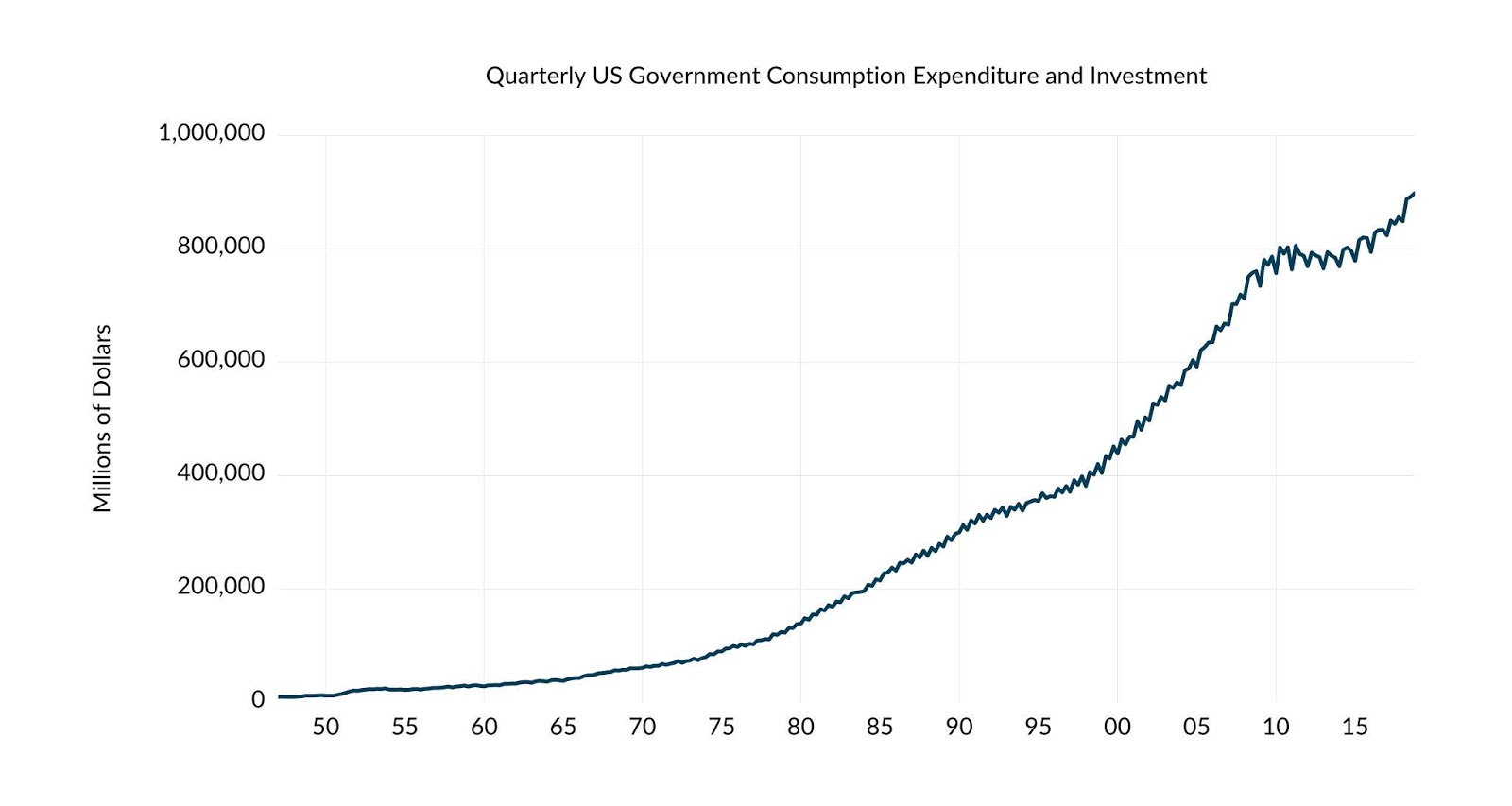Figure 5: Time Series Plot of USCONS

A visual analysis indicates data is trending with very prominent seasonal effects. To determine statistically whether these seasonal effects exhibit unit roots, we click on View/Unit Root Tests/Seasonal Unit Root Tests... to open the seasonal unit root test window.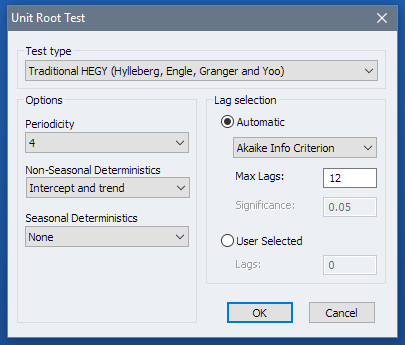Figure 6: HEGY Test Dialog

We will start with the HEGY test, which is the default test. Here, EViews has already filled out the periodicity with 4 to match the cyclicality of the data. Nevertheless, if you wish to test the data under a different periodicity, you may manually adjust this to one of the following supported values: 2, 4, 5, 6, 7, 12. Since our data is trending, we will change the Non-Seasonal deterministics dropdown from None to Intercept and trend and leave the Seasonal Deterministics dropdown unchanged.

As discussed earlier, in case of serially correlated errors, the HEGY test can be augmented by lags of the dependent variable added as additional regressors to the HEGY regression. To determine the precise number of lags to add, EViews offers both automatic and manual methods. The default is automatic lag selection with Akaike Information Criterion and maximum of 12 lags. The details can be changed of course, or, if automatic selection is undesired, a User Selected value can be specified. We will stick with the defaults. Hit OK.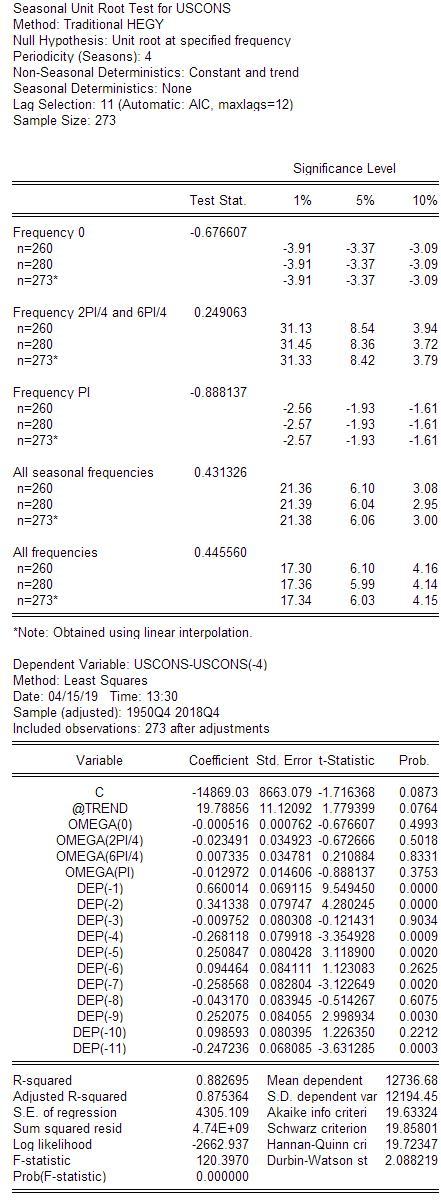Figure 7: HEGY Test Output

Looking at the output, EViews provides a table, the top portion of which summarizes the testing procedure, whereas the lower summarizes the regression output upon which the test is conducted. In particular, EViews computes the HEGY test statistic for each of the 0, harmonic pairs, and $\pi$ frequencies, in addition to the joint test for all seasonal frequencies -- a joint test for all frequencies other than 0 -- and a joint test for all frequencies including the frequency 0. As in traditional unit root tests, the null hypothesis postulates the existence of a unit root at the seasonal frequencies under consideration and rejection of the null requires the absolute value of the test statistic to exceed the absolute value of a critical value associated with the limiting distribution. In this regard, EViews summarizes the 1\%, 5\%, and 10\% critical values derived from simulation for sample sizes ranging from 20 to 480 in intervals of 20. To adjust for the actual sample size used in the HEGY regression, EViews also offers an interpolated version of the critical values. Here, it is clear that we will not reject the null hypothesis at any of the individual or harmonic pair frequencies, nor at the two joint tests. The overwhelming conclusion is that USCONS exhibits a unit root at each of the quarterly spectral frequencies individually and jointly.

Consider next the CH test applied to the same data. To bring up the CH test options, from the series object, once again click on View/Unit Root Tests/Seasonal Unit Root Tests... and under the Test type dropdown, select Canova and Hansen. As before, we will leave the Periodicity unchanged and will change the Non-Seasonal Deterministics to Intercept and trend. Note here that the traditional Canova and Hansen (1995) paper does not allow for the inclusion of deterministic trends. However, as noted in Busetti and Harvey (2003), we can relax the conditions of CH by showing that the distribution is unaffected when a deterministic trend is included in the model''.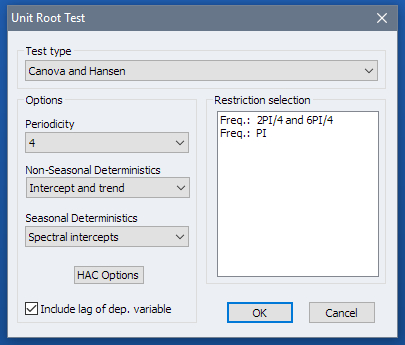Figure 8: CH Test Dialog

Next, change the Seasonal Deterministics dropdown from Seasonal dummies to Seasonal intercepts. Notice that when we do this the Restriction selection box changes to reflect that restrictions are no longer on seasonal dummies, but on seasonal intercepts. Note that we can multi-select which frequencies we would like to test. This is equivalent to specifying the entries of the matrix $\pmb{A}_{1}$ we considered earlier. If no restrictions are selected, which is the default, then EViews will test all available restrictions. Here we will not select anything.

We will also leave the Include lag of dep. variable untouched. As noted in Canova and Hansen (1995), the inclusion of a lagged dependent variable in the CH regression will reduce this serial correlation (we can think of this as a form of pre-whitening), yet not pose a danger of extracting a seasonal root''. At last, note the HAC Options button which opens a set of options associated with how the long-run variance is computed and gives users the option to customize which kernel and bandwidths are used, and whether further residual whitening is desired. We stick with default values and simply click on OK to execute the test.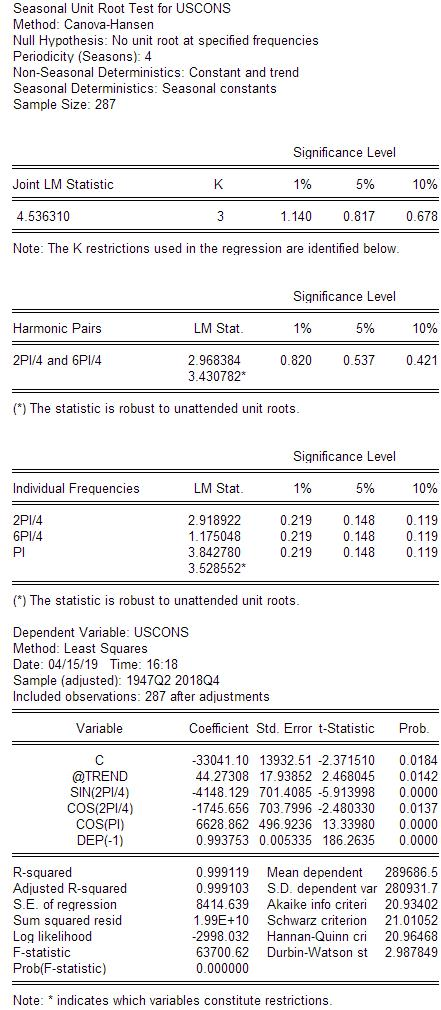Figure 9: CH Test Output

Turning to the output, EViews divides the analysis into four sections. The first is a table summarizing the joint test for all elements in $\pmb{A}_{1}$. In the example at hand, we have 3 restrictions -- 2 associated with the harmonic pair $(\frac{\pi}{2}, \frac{3\pi}{2})$ , and one associated with the frequency $\pi$. Since the null hypothesis is that no unit root exists at the specified frequencies and the test statistic 4.53631 is larger than either of the 1\%, 5\%, or 10\% critical values, we conclude that the joint test rejects the null hypothesis.

The next table presents a detailed look at the harmonic pair test. Although we did not explicitly ask for this test, EViews presents a breakdown of the joint test requested into its constituent restrictions. These are harmonic pair tests in which the restriction matrix $\pmb{A}_{1}$ would be $S\times 2$. In this case, the test for no seasonal unit root at the harmonic pair is 2.968384 which is clearly larger than any of the critical values associated with the limiting distribution. In other words, we reject the null and conclude that there's evidence of a unit root at the harmonic pair frequencies. Notice also that in addition to the CH test statistic EViews also offers an additional test statistic marked by an asterisk for differentiation. This is in fact the test statistic that corresponds to the Taylor (2003) version of the CH test robustified to the possible violation of the maintained hypothesis $H_{M}$ discussed earlier.

The table beneath the harmonic pair tests is a table summarizing CH tests corresponding to the individual breakdown of all frequencies under consideration. In other words, these are individual tests in which the restriction matrix $\pmb{A}_{1}$ would be $S\times 1$. Since the frequency $\pi$ was requested as part of the joint test, it is reported here. Clearly, with the test statistic equaling 3.842780, we reject the null hypothesis and conclude in favor of evidence supporting the existence of a unit root at the frequency $\pi$. As before, note here that below the test statistic associated with the $\pi$ frequency is an additional statistic differentiated by an asterisk. This, as before, is the Taylor (2003) version of the CH test robustified to unattended unit roots.

At last, the final table presents the CH regression. The residuals from this regression are used in the computation of the CH test statistics.

### Conclusion

In this entry we gave a brief introduction into the subject of seasonal unit root tests. We highlighted the need to distinguish between deterministic and stochastic cyclicality and discussed several statistical methods designed to do so. Among these, our focus was on the HEGY tests, which is effectively an extension of the ADF test in the direction of non-zero seasonal frequencies, and the CH test, which is the analogue of the KPSS test in the direction of non-zero seasonal frequencies. We also looked at some of the mathematical details which underly these methods. At last, we closed with a brief application of both tests to the US Government consumption expenditure and investment data, sampled quarterly from 1947 to 2018. Both tests overwhelmingly supported evidence of unit roots at both individual and joint frequencies.

### References

 1 Fabio Busetti and AM Robert Taylor. Testing against stochastic trend and seasonality in the presence of unattended breaks and unit roots. Journal of Econometrics, 117(1):21--53, 2003. [ bib ] 2 Fabio Busetti and Andrew Harvey. Seasonality tests. Journal of Business & Economic Statistics, 21(3):420--436, 2003. [ bib ] 3 Fabio Canova and Bruce E Hansen. Are seasonal patterns constant over time? a test for seasonal stability. Journal of Business & Economic Statistics, 13(3):237--252, 1995. [ bib ] 4 Svend Hylleberg, Robert F Engle, Clive WJ Granger, and Byung Sam Yoo. Seasonal integration and cointegration. Journal of econometrics, 44(1-2):215--238, 1990. [ bib ] 5 Denis Kwiatkowski, Peter CB Phillips, Peter Schmidt, and Yongcheol Shin. Testing the null hypothesis of stationarity against the alternative of a unit root: How sure are we that economic time series have a unit root? Journal of econometrics, 54(1-3):159--178, 1992. [ bib ] 6 Richard J Smith and AM Robert Taylor. Likelihood ratio tests for seasonal unit roots. Journal of Time Series Analysis, 20(4):453--476, 1999. [ bib ] 7 Robert AM Taylor. Robust stationarity tests in seasonal time series processes. Journal of Business & Economic Statistics, 21(1):156--163, 2003. [ bib ] 8 AM Robert Taylor. Variance ratio tests of the seasonal unit root hypothesis. Journal of Econometrics, 124(1):33--54, 2005. [ bib ]

1.2.